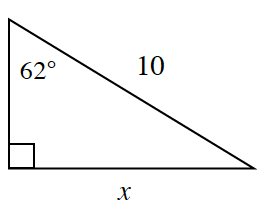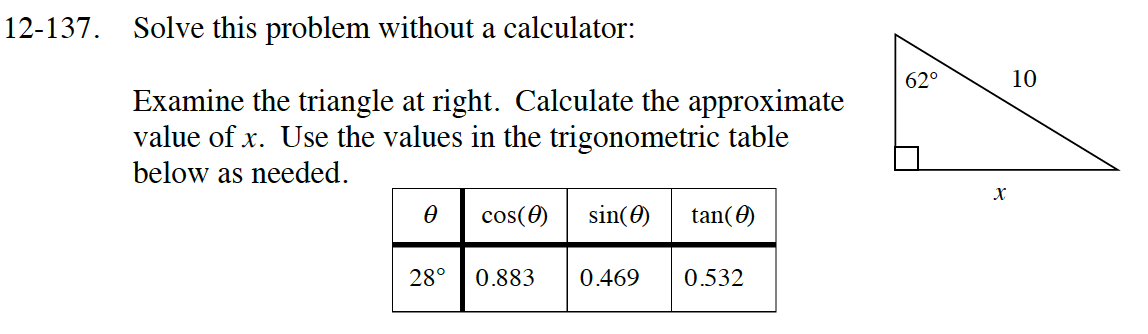### Home > INT2 > Chapter 12 > Lesson 12.2.4 > Problem12-137

12-137.
1.Solve this problem without a calculator:

Examine the triangle at right. Calculate the approximate value of x. Use the values in the trigonometric table below as needed. Homework Help ✎

2.  θ cos(θ) sin(θ) tan(θ) 28° 0.883 0.469 0.532$\text{Remember that sin }(\theta)=\frac{\text{opp.}}{\text{hyp.}}, \text{ cos }(\theta)=\frac{\text{adj.}}{\text{hyp.}}, \text{ and tan }(\theta)=\frac{\text{opp.}}{\text{adj.}}.$

How are sin(62) and cos(68) related?